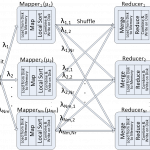## Posts

Showing posts from May, 2016

### FORK-JOIN QUEUE MODELING AND OPTIMAL SCHEDULING IN PARALLEL PROGRAMMING FRAMEWORKSFORK-JOIN QUEUE MODELING AND OPTIMAL SCHEDULING IN PARALLEL PROGRAMMING FRAMEWORKS ABSTRACT MapReduce framework is widely used to parallelize batch jobs since it exploits a high degree of multi-tasking to process them. However, it has been observed that when the number of servers increases, the map phase can take much longer than expected. This thesis analytically shows that the stochastic behavior of the servers has a negative effect on the completion time of a MapReduce job, and continuously increasing the number of servers without accurate scheduling can degrade the overall performance. We analytically model the map phase in terms of hardware, system, and application parameters to capture the effects of stragglers on the performance. Mean sojourn time (MST), the time needed to sync the completed tasks at a reducer, is introduced as a performance metric and mathematically formulated. Following that, we stochastically investigate the optimal task scheduling which leads to an equilibri…